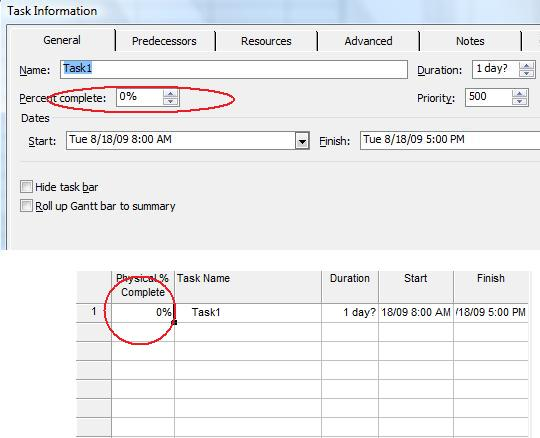# Percentage Complete Calculations

Contents
[ ]

## Percentages

The Task class exposes several properties used to calculate percentages:

• PercentComplete represents the completed percentage of a task’s duration (integer).
• PercentWorkComplete represents the completed percentage of a task’s work (integer).
• PhysicalPercentComplete represents the completed percentage as entered by a project manager (integer).

To see the physical percent complete in Microsoft Project:

1. On the Task Entry form, from the Insert menu, select Column.
2. Add the column.

To see the completed percentage in Microsoft Project on the Task Entry form, double-click the desired column:

A task’s completed percentage in Microsoft Project, and, below it, it’s physical completion percentage.### Getting Percentages in Aspose.Tasks

The following example shows how to get the percentages of work relating to tasks using Aspose.Tasks.